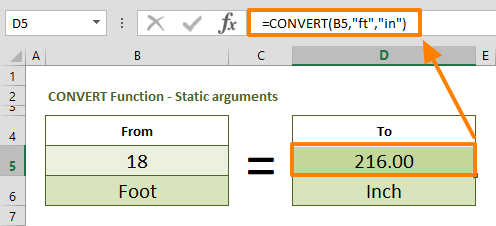Converting units can be time consuming when you rely on a separate tool or website to do it.​ In this guide, we are going to show you how to convert feet to inches and inches to feet in Excel.

## Manual approach

The first approach we are going to show you is doing the conversion manually. You can convert feet to inches using a simple math calculation. 1 foot is equal to 12 inches. Thus, you need to multiply the foot-value by 12 to get the same value in inches.

Let's say the cell C2 contains the foot-value. Use the formula below to find the inch-value.

=C2*12In a similar way, you can convert feet to inches. As you can see in the above example, dividing an inch-value by 12 returns the value in feet. If we assume that the cell C5 contains the inch-value, the conversion formula would be something like,

=C5/12

Now, let's take a look at more dynamic and relatively easy to remember approaches.

## Using the CONVERT Function

Another way to convert feet to inches is using the CONVERT function. The CONVERT function can convert a number from one measurement system to another. The best part is that the function is not limited to only length units. You can convert weight, distance, temperature and more units of measurements between each other.

You can find more information about the CONVERT function with more examples in our guide: Excel CONVERT Function

Briefly, you need to supply the value and both measurement types into the function. Excel accepts string abbreviations of the measurement types. In this example you can use,

• Foot: "ft"
• Inch: "in"

As a result, to convert the value in cell B5, you can use a formula like below:

=CONVERT(B5,"ft","in")To make the conversion in the opposite direction, simply switch the measurement arguments. The following formula converts the value from inches to feet.

=CONVERT(B5,"in","ft")## Another dynamic method

You can set cell references for unit arguments. This application allows you to control the from and to unit types. We combined the CONVERT and VLOOKUP functions to create a master formula which gets units to convert from a list or any other input type. With the help of the VLOOKUP function, we can use user-friendly names for unit type codes. For example, "feet" for "ft" and "inches" for "in".

The VLOOKUP function returns the corresponding unit type for the CONVERT function. Here is what the formula would look like:

=CONVERT(B11,VLOOKUP(B12,Length.Table,2,0),VLOOKUP(D12,Length.Table,2,0))

The cells B12 and D12 contains a dropdown list which contains length type of measurement units like "Feet" and "Inch" strings. The VLOOKUP function searches them in named range Length.Table and returns the corresponding value.